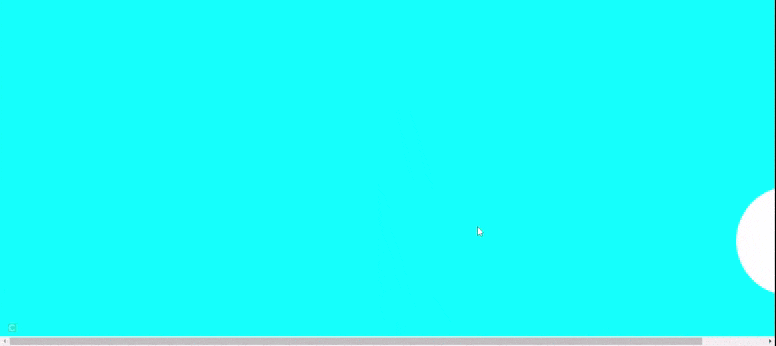# How to create a moving div using JavaScript ?

• Last Updated : 25 May, 2021

In this article, we will learn to create a moving HTML div using JavaScript. The div will move left to right using HTML, CSS, and JavaScript

Approach:

Hey geek! The constant emerging technologies in the world of web development always keeps the excitement for this subject through the roof. But before you tackle the big projects, we suggest you start by learning the basics. Kickstart your web development journey by learning JS concepts with our JavaScript Course. Now at it's lowest price ever!

1. We have to create an HTML div and add some CSS to the div using a class ball.
2.  In CSS, we add some background-color to the body and give some height, width, and color to the div.
3. Now we will add margin-left to the div using JavaScript. So it will move left to right.
4. In JavaScript, we grab the div using the id name. And after some interval of time, we will add margin-left to the div.

HTML code:

## HTML

 ```<``html` `lang``=``"en"``>``  ``<``head``>``    ``<``meta` `charset``=``"UTF-8"` `/>``    ``<``meta` `http-equiv``=``"X-UA-Compatible"` `          ``content``=``"IE=edge"` `/>``    ``<``meta` `name``=``"viewport"` `          ``content``=``"width=device-width, initial-scale=1.0"` `/>`` ` `    ``<``style``>``      ``body {``        ``background-color: aqua;``        ``display: flex;``        ``align-items: center;``      ``}`` ` `      ``.ball {``        ``height: 12rem;``        ``width: 12rem;``        ``background-color: white;``        ``border-radius: 50%;``        ``margin-top: 20rem;``      ``}``    ````  ````  ``<``body``>``    ``<``div` `class``=``"container"``>``      ``<``div` `class``=``"ball"` `id``=``"ballID"``>``    ````    ``<``script``>``      ``let ball = document.getElementById("ballID");`` ` `      ``var myVar = setInterval(spostaDiv, 90);``      ``var margin = 0;`` ` `      ``let l = window.screen.width;``      ``let w = 1300;`` ` `      ``function spostaDiv() {``        ``console.log(w);``        ``if (margin == w) {``          ``margin = 0 + "px";``        ``} else {``          ``ball.style.marginLeft = margin + "px";``        ``}``        ``margin += 10;``      ``}``    ````  `````

Output:My Personal Notes arrow_drop_up